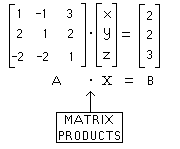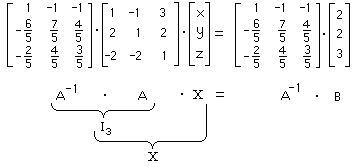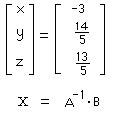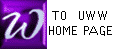MATRIX INVERSE METHOD
for solving a system of equations

See the similar discussion in our text, Rolf, on Pages 165-167 ;
the following continues an earlier discussion.

 We start with a system of equations (right), to be solved. The number of variables must equal the number of equations, both equal to 3 here:
 x - y + 3z = 2 2x + y + 2z = 2 -2x - 2y + z = 3

On Prof. McFarland's tests, you would be asked to solve the above problem

"by the matrix inverse method" in three distinct steps, as follows :


 Write the given system (above) as a single matrix equation:

Capital letter variables represent the matrices (not numbers) which sit directly above them. Hence, the above equation is a matrix equation. Notice how the matrix entries correspond to numbers in the original system of 3 equationsSolve the matrix equation obtained in step  above; i.e., find X.
In the MATRIX INVERSE METHOD (unlike Gauss/Jordan), we solve for the matrix variable X by left-multiplying both sides of the above matrix equation (AX=B) by A-1. Typically, A-1 is calculated as a separate exercize ; otherwise, we must pause here to calculate A-1.The left side (above) is easy to calculate because an identity matrix I 3 appears. Note: A-1 is on the LEFT side of both products.
Step  is now completed by finding A-1. B ===>Using  above, write the solution to the original system:
original system
 x - y + 3z = 2 2x + y + 2z = 2 -2x - 2y + z = 3

solution to original system
 x = -3 y = 145 z = 135